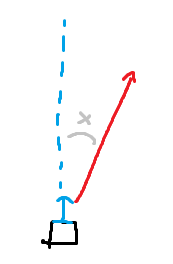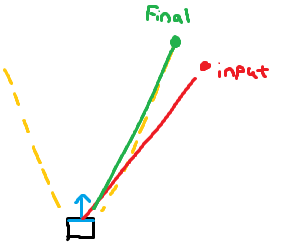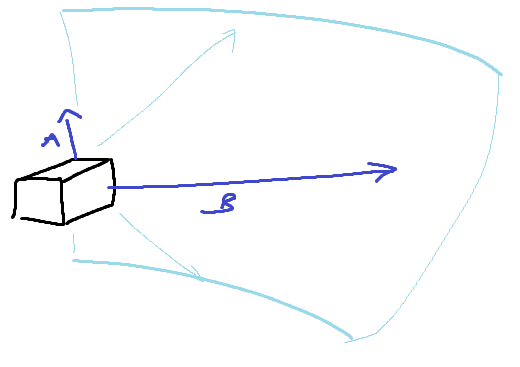# Check if angle is in bounds?

Hey I’d like to make something that checks if a certain angle facing out from a point is within appropriate bounds.

I’m not sure how to even begin approaching this, so I’m curious if there’s some kind of simple way to do this.
If there was a way to reduce the angle between a part’s forward facing direction (unit) and a new angle in relation to that (view below) to a single number or valueIt should then be easy to do something where you can get a true or false on something based on whatever “angle boundary” you want like so:

This is for cannons in a game I’m making. Curious what solutions you guys would use for this, because anything I can work up right now is extremely sloppy.
Also, since I’m working with cannons, if it’s possible to get a returned value on “closest in boundary direction” that would be an extra challengeIs Vector3:Dot perfect for this?

Found something kinda relevant: When do I have to use Vector:dot() or Vektror:cross() and what exactly can I use them for in 3D space (workspace)?

Dot Vector is what your exactly looking for as it returns a scalar between two positions which can be converted to an angle if im not wrong with math.acos

1 Like

For sure
However, finding the nearest angle that is in bounds seems to be trickier than I thought

EDIT:

Might have found what I was looking for

You can use the dot product another way. If you had two unit vectors `a` and `b`, the closer `a:Dot(b)` (or `b:Dot(a)` because dot product commutative) gets to 1, the more they’re on the same direction. If they’re not looking in opposite directions, the value should be between 1 and 0.

What you can do is, first check the value of the dot product for a vector that’s exactly on the edge of the angle boundary, say a 60 degrees angle. That would be `a:Dot(Vector3.new(0,0,math.cos(math.rad(x))))`, where `x` is your angle, 60 degrees in our case, and `a` is your part’s lookVector. Then you simply check if `a:Dot((b-a).unit)` is `<=` less or equal to `a:Dot(Vector3.new(0,0,math.cos(math.rad(x))))`. `(b-a)` is the vector between your part’s lookVector and the position to check if in bounds, `(b-a).unit` is the direction of that, because we need both `a` and the second vector to be unit vectors.

``````local part = --your part
local lookVector = part.CFrame.lookVector
local angle = 60
local threshold = lookVector:Dot(Vector3.new(math.cos(angle),0,0))
local position = --position inside or outside of bounds
if lookVector:Dot((lookVector - position).unit) <= threshold then
--something
end
``````

Yeah using two units seems to be the best approach
This is a lot easier with say only left/ right angles, but I’m trying to think of a way that covers the up/ down trajectory too without creating another pair of units.I’m still completely dumbfounded how to do the clamping without a ton of checks and extra units
Bear in mind I won’t be doing this every frame so I can afford a bit of a complex calc

My big challenge right now is converting the “up down” angle of an out of bounds trajectory into in bounds